Test: Dimensional Geometry - 5

# Test: Dimensional Geometry - 5

Test Description

## 20 Questions MCQ Test Topic-wise Tests & Solved Examples for IIT JAM Mathematics | Test: Dimensional Geometry - 5

Test: Dimensional Geometry - 5 for Mathematics 2023 is part of Topic-wise Tests & Solved Examples for IIT JAM Mathematics preparation. The Test: Dimensional Geometry - 5 questions and answers have been prepared according to the Mathematics exam syllabus.The Test: Dimensional Geometry - 5 MCQs are made for Mathematics 2023 Exam. Find important definitions, questions, notes, meanings, examples, exercises, MCQs and online tests for Test: Dimensional Geometry - 5 below.
Solutions of Test: Dimensional Geometry - 5 questions in English are available as part of our Topic-wise Tests & Solved Examples for IIT JAM Mathematics for Mathematics & Test: Dimensional Geometry - 5 solutions in Hindi for Topic-wise Tests & Solved Examples for IIT JAM Mathematics course. Download more important topics, notes, lectures and mock test series for Mathematics Exam by signing up for free. Attempt Test: Dimensional Geometry - 5 | 20 questions in 60 minutes | Mock test for Mathematics preparation | Free important questions MCQ to study Topic-wise Tests & Solved Examples for IIT JAM Mathematics for Mathematics Exam | Download free PDF with solutions
 1 Crore+ students have signed up on EduRev. Have you?
Test: Dimensional Geometry - 5 - Question 1

### The line joining (3, -1) and (2, 3)

Detailed Solution for Test: Dimensional Geometry - 5 - Question 1

Proof: We have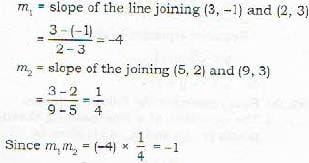therefore the line joining (3, -1) and (2, 3) is perpendicular to the line joining (5, 2) and (9, 3).

Test: Dimensional Geometry - 5 - Question 2

### The equation of the bisector of the angle between the lines 3x - 4y + 7 = 0 and 12x - 3y -8 =0 , in w hich the origin lines, is given by

Detailed Solution for Test: Dimensional Geometry - 5 - Question 2

Equations of Bisectors of the Angles between two non-parallel lines.
Let the straight lines
Ax+ By + C = 0 and
A'x + B'y + C = 0
be non-parallel. Then the bisectors are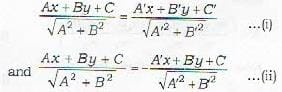Rules:
1. if C > 0, C' > 0 and AA' + BB' > 0, then (i) is the equation of obtuse - angle bisector (so the equation (ii) gives the acute - angle bisector).
2. If C > 0, C' > 0 and AA' * BB' < 0, then (i) is the equation of acute - angle bisector (so (ii) gives the obtuse - angle bisector)
3. If C and C' are of the same sign (cither both positive or both negative), then (i) is the bisector of that angle (acute or obtuse) in which the origin lies.
In Problem 42, the given equations are
3x-4y + 7= 0 and
12x - 5y = 8 = 0
Since C and C' are of opposite sign, therefore the bisector of the angle between (iii) and (iv), in which the origin lies in given by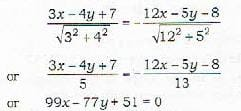Test: Dimensional Geometry - 5 - Question 3

### The line joining the origin to the point of intersection of 2x + 5y- 4 = 0 and 3x-2y+ 2 = 0 is given by

Detailed Solution for Test: Dimensional Geometry - 5 - Question 3

The given straight lines are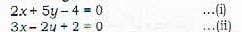Their point of intersection is obtained on solving equations (i) and (ii) and is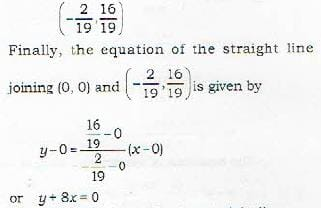Test: Dimensional Geometry - 5 - Question 4

The area of the triangle formed by the lines y -x = 0, y + x = 0, x - c = 0 is given by

Detailed Solution for Test: Dimensional Geometry - 5 - Question 4

The triangle is formed bv the straight lines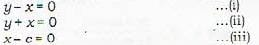Their points of intersection are the vertices of the triangle and are (0, 0), (c, c) and (c, -c) The required area Δ is given by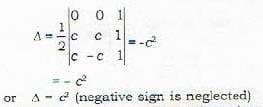Test: Dimensional Geometry - 5 - Question 5

A homogeneous equation of nth degree represents

Detailed Solution for Test: Dimensional Geometry - 5 - Question 5

A homogeneous equation of nth degree in x and y is a0xn + a1xn-1 + a2xn-2+ a3xn-3 .....+ an-1xyn-1+ anyn=0 and it represents at most n straight lines passing through the origin.

Test: Dimensional Geometry - 5 - Question 6

The angle between the straight lines represented by the equation Ax2 + 2Bxy + Cy2 = 0 is given by

Test: Dimensional Geometry - 5 - Question 7

The lines represented by the equation Ax2 + 2Bxy + Cy2 = 0 are real, coincident or imaginary according as B2 - AC is

Detailed Solution for Test: Dimensional Geometry - 5 - Question 7

The lines are represented by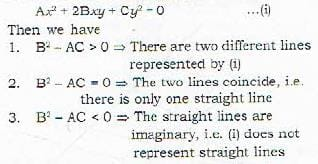Test: Dimensional Geometry - 5 - Question 8

The lines represented by the equation Ax2 + 2Bxy + Cy2 = 0 are perpendicular if

Detailed Solution for Test: Dimensional Geometry - 5 - Question 8

The angle φ between the lines is given by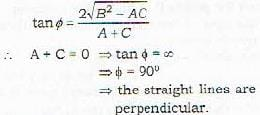Test: Dimensional Geometry - 5 - Question 9

The general equation of second degree ax2 + 2hxy + by2 + 2gx + 2fy + c = 0 may represent a pair of straight lines if

Detailed Solution for Test: Dimensional Geometry - 5 - Question 9

The condition that the equation ax2 + 2hxy + by2 + 2gx + 2fy + c = 0 represents a pair of straight lines is that af2+bg2+ch2+2jgh-abc=0

Test: Dimensional Geometry - 5 - Question 10

The lines joining ihe origin to the points of intersection of 2x2 + 3xy - 4x + 1 = 0 and 3x + y - 1 = 0 are given by

Detailed Solution for Test: Dimensional Geometry - 5 - Question 10

The required equation of lines is obtained bymaking equation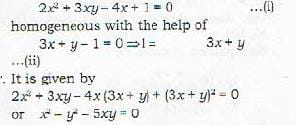Test: Dimensional Geometry - 5 - Question 11

The area of the triangle ABC formed by the intercepts OA a, OB = h, OC = con the coordinate axes respectively by a plane is given by

Detailed Solution for Test: Dimensional Geometry - 5 - Question 11

If Ax, Ay, Az be the areas of projection of an area A on the three coordinate planes then A2=Ax2 + Ay2 + Az
we have to find the area A of triangle ABC Ax =  the projection of A on yz plane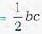Ay = the projection of A on zx plane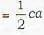Az = the projection of A on xy plane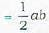∴ A2=Ax2 + Ay2 + Az2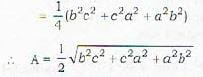Test: Dimensional Geometry - 5 - Question 12

If two pairs of opposite edges of a tetrahedron art; at right angles, then the third pair

Detailed Solution for Test: Dimensional Geometry - 5 - Question 12

Let the coordinates of the vertices O, A, B, C o f tetrahedron be (α, β, γ), (a, 0, 0), (0, b, 0) and (0. 0, c) respectively. Then it is given that
OA is perpendicular to BC
and OB is perpendicular to CA
To prove that
OC is perpendicular to AB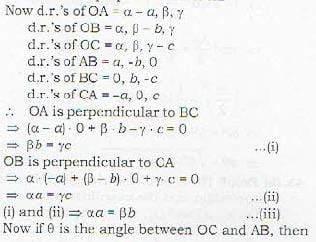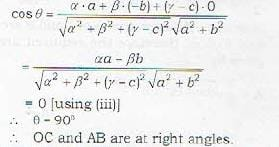Test: Dimensional Geometry - 5 - Question 13

If the pairs of opposite edges of a tetrahedron OABC are at right angles, then

Test: Dimensional Geometry - 5 - Question 14

How many arbitrary constants are there in the equation of a plane?

Detailed Solution for Test: Dimensional Geometry - 5 - Question 14

The general equation of first degree in x, y, z namely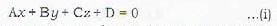represents a plane, where at least one of A, B and C is non-zero.
Remark: 1. Let A ≠ 0. Then equation (i)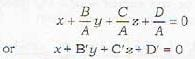Therefore the general equation of a plane involves three independent (essential) arbitrary constant.
Hence if three independent conditions are given then the plane can be determined completely.
Remark : 2. If D = 0 then the plane will pass through the origin.

Test: Dimensional Geometry - 5 - Question 15

The equation of which of the following degree in x, y, z represents a plane

Test: Dimensional Geometry - 5 - Question 16

Which one of the following is the equation of the plane which meets the coordinale axes in A,B,C such that the centroid of the triangle ARC is (a,b,c)?

Detailed Solution for Test: Dimensional Geometry - 5 - Question 16

Let the coordinates of A, B and C be (a, 0, 0), (0, β, γ) and (0,0, y) respectively. Then the coordinates of the centroid of ΔABC is given by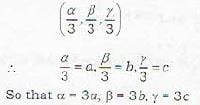In other words, the coordinates of A, B and C are
(3a, 0, O), (0, 3b, 0) and (0, 0, 3c) respectively.
Verify that the secoordinates of A, B and C satisfy equation in statement (c)

Test: Dimensional Geometry - 5 - Question 17

If l, m, n are the direction of the normal to the plane and p be the perpendicular distance from the origin on it, then the equation of the plane is of the type

Detailed Solution for Test: Dimensional Geometry - 5 - Question 17

OP is perpendicular on the plane ABC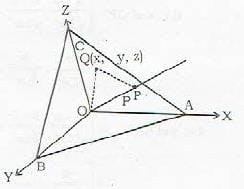∴ OP = p and d.c.'s of OP are [1, m, n]
Let Q(x, y, z) be an arbitrary point on the plane.
Then
OP is the projection of OQ on OP.
⇒ OP = I(x- 0) + m(y- 0) + n(z- 0)
⇒ lx + my + nz = p
This is the required equation of the plane in normal form.

Test: Dimensional Geometry - 5 - Question 18

The equation of the plane passing through the point A(a, b, c) and perpendicular to OA (O being origin) is given by

Detailed Solution for Test: Dimensional Geometry - 5 - Question 18

Proof: OA is normal to the plane.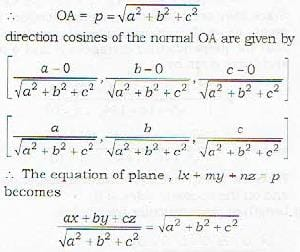or ax + by + cz = a2 + b2 + c2 (statement(al))
Further, Let P(x, y, z) be any point on the plane.
Then OA is perpendicular to PA.
=> (x-a)a + (y- b)b+(z- c)c=0
(This is statement (b))
.*. both (a) and (b) are correct.

Test: Dimensional Geometry - 5 - Question 19

The equation of the plane passing through (2, -3, 1) and perpendicular to the line joining the points (3, 4. -1) and (2, -1, 5) is given by

Detailed Solution for Test: Dimensional Geometry - 5 - Question 19

Let P(x, y, z) be an arbitrary point on the plane. Then the line PA joining the points P(x, y, z) and A(2, -3, 1) lies on the plane and the d.r.’s of PA are
x - 2, y + 3, z- 1
Further, the direction ratios of the line joining points B(3, 4. - 1 ) and C(2, 1,5) are
3 - 2 , 4 - ( - 1 ),- 1 -5
i.e. 1, 5, -6
Since BC is perpendicular to the plane and hence is perpendicular to line PA, therefore
( x - 2) 1 + (y + 3) 5 + ( z - 1) (- 6 ) = 0
or x + 5y - 6z + 19 = 0

Test: Dimensional Geometry - 5 - Question 20

The equation of the plane through the points (2, 3, 1) and (-4. 5. 3) and parallel to X - axis is given by

Detailed Solution for Test: Dimensional Geometry - 5 - Question 20

The plane is parallel' to x-axis. Therefore, it is perpendicular to yzplane.
Hence its equation should be of the form
By Cz + D = 0
Since, the plane passes through (2, 3, 1) and (4, -5, 3), therefore.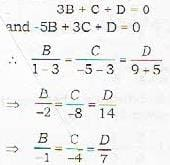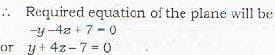## Topic-wise Tests & Solved Examples for IIT JAM Mathematics

27 docs|150 tests
 Use Code STAYHOME200 and get INR 200 additional OFF Use Coupon Code
Information about Test: Dimensional Geometry - 5 Page
In this test you can find the Exam questions for Test: Dimensional Geometry - 5 solved & explained in the simplest way possible. Besides giving Questions and answers for Test: Dimensional Geometry - 5, EduRev gives you an ample number of Online tests for practice

## Topic-wise Tests & Solved Examples for IIT JAM Mathematics

27 docs|150 tests